JEE  >  Test: Vector Algebra- 1

# Test: Vector Algebra- 1

Test Description

## 25 Questions MCQ Test Mathematics (Maths) Class 12 | Test: Vector Algebra- 1

Test: Vector Algebra- 1 for JEE 2022 is part of Mathematics (Maths) Class 12 preparation. The Test: Vector Algebra- 1 questions and answers have been prepared according to the JEE exam syllabus.The Test: Vector Algebra- 1 MCQs are made for JEE 2022 Exam. Find important definitions, questions, notes, meanings, examples, exercises, MCQs and online tests for Test: Vector Algebra- 1 below.
Solutions of Test: Vector Algebra- 1 questions in English are available as part of our Mathematics (Maths) Class 12 for JEE & Test: Vector Algebra- 1 solutions in Hindi for Mathematics (Maths) Class 12 course. Download more important topics, notes, lectures and mock test series for JEE Exam by signing up for free. Attempt Test: Vector Algebra- 1 | 25 questions in 25 minutes | Mock test for JEE preparation | Free important questions MCQ to study Mathematics (Maths) Class 12 for JEE Exam | Download free PDF with solutions
 1 Crore+ students have signed up on EduRev. Have you?
Test: Vector Algebra- 1 - Question 1

### Vector has

Detailed Solution for Test: Vector Algebra- 1 - Question 1

A vector has both magnitude as well as direction.

Test: Vector Algebra- 1 - Question 2

### Correct form of distributive law is

Detailed Solution for Test: Vector Algebra- 1 - Question 2

Distributive law is given by :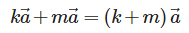Test: Vector Algebra- 1 - Question 3

### Magnitude of the vector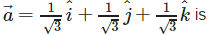Detailed Solution for Test: Vector Algebra- 1 - Question 3

We have :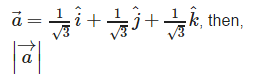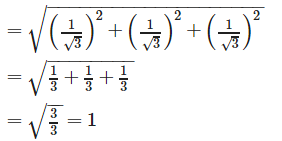Test: Vector Algebra- 1 - Question 4

Find the unit vector in the direction of vector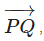where P and Q are the points (1, 2, 3) and (4, 5, 6), respectively

Detailed Solution for Test: Vector Algebra- 1 - Question 4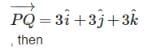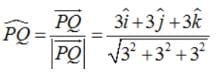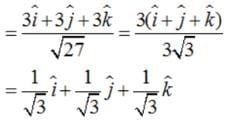Test: Vector Algebra- 1 - Question 5

If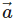is a non zero vector of magnitude ‘a’ and λ a non zero scalar, then λis a unit vector if

Detailed Solution for Test: Vector Algebra- 1 - Question 5

λis a unit vector if and only ifis equal to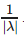Test: Vector Algebra- 1 - Question 6

Find the values of x and y so that the vectors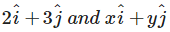are equal

Detailed Solution for Test: Vector Algebra- 1 - Question 6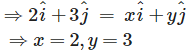Test: Vector Algebra- 1 - Question 7

If P1(x1, y1, z1) and P2(x2, y2, z2) are any two points, then the vector joining P1 and P2is the vector P1P2. Magnitude of the vector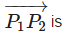Detailed Solution for Test: Vector Algebra- 1 - Question 7

If P1(x1, y1, z1) and P2(x2, y2, z2) are any two points, then the vector joining P1 and P2is the vector P1P2, then ;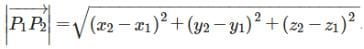Test: Vector Algebra- 1 - Question 8

Find the scalar and vector components of the vector with initial point (2, 1) and terminal point (– 5, 7).

Detailed Solution for Test: Vector Algebra- 1 - Question 8

The scalar and vector components of the vector with initial point (2, 1) and terminal point (– 5, 7) is given by : (- 5 – 2) i.e. – 7 and (7 – 1) i.e. 6. Therefore, the scalar components are – 7 and 6 .,and vector components are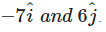Test: Vector Algebra- 1 - Question 9

Find a vector in the direction of the vector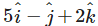which has a magnitude of 8 units

Detailed Solution for Test: Vector Algebra- 1 - Question 9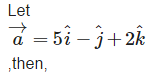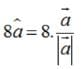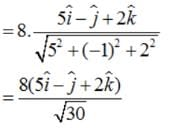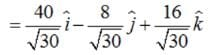Test: Vector Algebra- 1 - Question 10

Find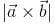, if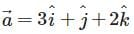and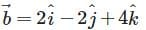Detailed Solution for Test: Vector Algebra- 1 - Question 10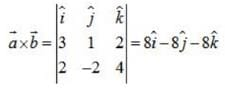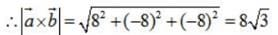Test: Vector Algebra- 1 - Question 11

Direction angles are angles

Detailed Solution for Test: Vector Algebra- 1 - Question 11

α,β,γ are the angles which the position vector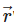makes with the positive x-axis ,y-axis and z-axis respectively are called direction angles.

Test: Vector Algebra- 1 - Question 12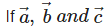are any three vectors then the correct expression for distributivity of scalar product over addition is

Detailed Solution for Test: Vector Algebra- 1 - Question 12are any three vectors then the correct expression for distributivity of scalar product over addition is :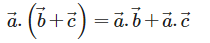Test: Vector Algebra- 1 - Question 13

Find the values of x and y so that the vectorsDetailed Solution for Test: Vector Algebra- 1 - Question 13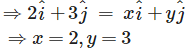Test: Vector Algebra- 1 - Question 14

Find the direction cosines of the vector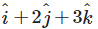Detailed Solution for Test: Vector Algebra- 1 - Question 14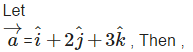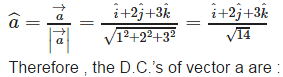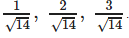Test: Vector Algebra- 1 - Question 15

Find a unit vector perpendicular to each of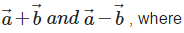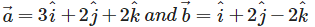Detailed Solution for Test: Vector Algebra- 1 - Question 15

It is given that: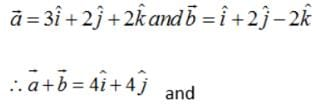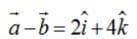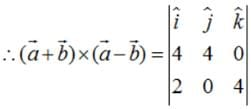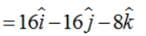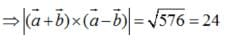Therefore, the unit vector perpendicular to both the vectors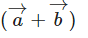and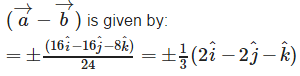Test: Vector Algebra- 1 - Question 16

Direction cosines

Detailed Solution for Test: Vector Algebra- 1 - Question 16

Cosines of the angles α,β,γ are called direction cosines.

Test: Vector Algebra- 1 - Question 17

Magnitude of the vector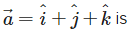Detailed Solution for Test: Vector Algebra- 1 - Question 17

We have :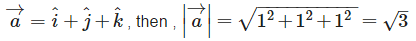Test: Vector Algebra- 1 - Question 18

Find the sum of the vectors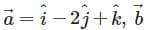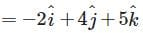and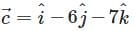Detailed Solution for Test: Vector Algebra- 1 - Question 18

We have: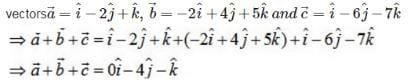Test: Vector Algebra- 1 - Question 19

Find the direction cosines of the vector joining the points A(1, 2, –3) and B(–1, –2, 1), directed from A to B.

Detailed Solution for Test: Vector Algebra- 1 - Question 19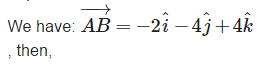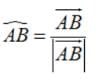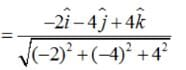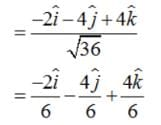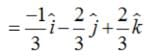Therefore, the D.C.’s of vector AB are given by: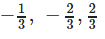Test: Vector Algebra- 1 - Question 20

If a unit vector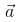makes angles π/3 with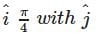and an acute angle θ with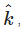then find θ

Detailed Solution for Test: Vector Algebra- 1 - Question 20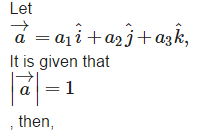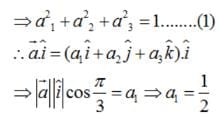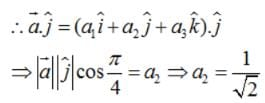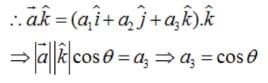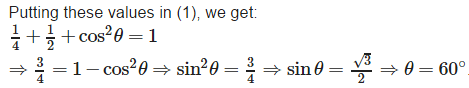Test: Vector Algebra- 1 - Question 21

If l, m and n are direction cosines of the position vector OP the coordinates of P are

Detailed Solution for Test: Vector Algebra- 1 - Question 21

If l , m and n are the direction cosines of vector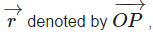then , the coordinates of point P are given by : lr ,mr and nr respectively.

Test: Vector Algebra- 1 - Question 22

Unit vectors along the axes OX, OY and OZ are denoted by

Detailed Solution for Test: Vector Algebra- 1 - Question 22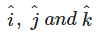represents the unit vectors along the co ordinate axis i.e. OX ,OY and OZ respectively.

Test: Vector Algebra- 1 - Question 23

Write down a unit vector in XY-plane, making an angle of 30° with the positive direction of x-axis.

Detailed Solution for Test: Vector Algebra- 1 - Question 23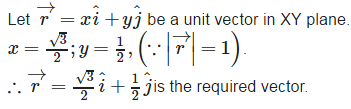Test: Vector Algebra- 1 - Question 24

Find the angle between two vectors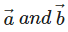with magnitudes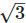and 2, respectively, having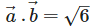Detailed Solution for Test: Vector Algebra- 1 - Question 24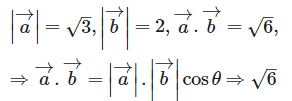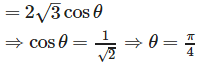Test: Vector Algebra- 1 - Question 25

If a unit vector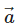makes angles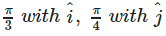and an acute angle θ with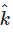, then the components ofare

Detailed Solution for Test: Vector Algebra- 1 - Question 25

Let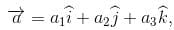It is given that left|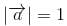, then ,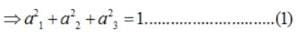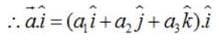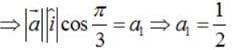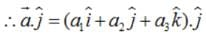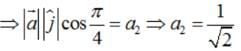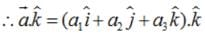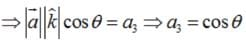Putting these values in (1) , we get :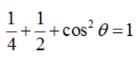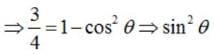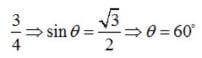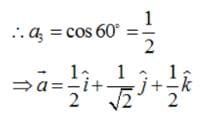## Mathematics (Maths) Class 12

209 videos|209 docs|139 tests
 Use Code STAYHOME200 and get INR 200 additional OFF Use Coupon Code
Information about Test: Vector Algebra- 1 Page
In this test you can find the Exam questions for Test: Vector Algebra- 1 solved & explained in the simplest way possible. Besides giving Questions and answers for Test: Vector Algebra- 1, EduRev gives you an ample number of Online tests for practice

## Mathematics (Maths) Class 12

209 videos|209 docs|139 tests